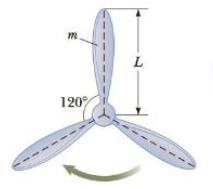Chapter 8, Problem 75AP

Chapter
Section
Textbook Problem

Additional ProblemsA typical propeller of a turbine used to generate electricity from the wind consists of three blades as in Figure P8.75. Each blade has a length of L = 35 in and a mass of m = 420 kg. The propeller rotates at the rate of 25 rev/min. (a) Convert the angular speed of the propeller to units of rad/s. Find (b) the moment of inertia of the propeller about the axis of rotation and (c) the total kinetic, energy of the propeller.Figure P8.75

(a)

To determine
The conversion of angular speed of the propeller to units of rad/s .

Explanation

The one revolution per minute such that 1rev/min is equal to 2πrad/60s and using this, the conversion of angular speed of 25rev/min to rad/s is ω=(25revmin

(b)

To determine
The moment of inertia of the propeller about the axis of rotation.

(c)

To determine
The total kinetic energy of the propeller.

Still sussing out bartleby?

Check out a sample textbook solution.

See a sample solution

The Solution to Your Study Problems

Bartleby provides explanations to thousands of textbook problems written by our experts, many with advanced degrees!

Get Started